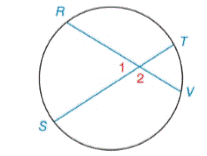Chapter 6.2, Problem 14EElementary Geometry For College St...

7th Edition
Alexander + 2 others
ISBN: 9781337614085

Solutions

Chapter
SectionElementary Geometry For College St...

7th Edition
Alexander + 2 others
ISBN: 9781337614085
Textbook Problem

Given: m ∠ 1 = 63 ° m R S ⌢ = 3 x + 6 m V T ⌢ = x Find: m R S ⌢Exercises 14, 15

To determine

To find:

mRS when m1=63°, mRS=3x+6,mVT=x

Explanation

Theorem:

The measure of an angle formed by two chords that intersect within a circle is one-half the sum of the measures of the arcs intercepted by the angle and its vertical angle.

Calculation:

Given,

mRS when m1=63°, mRS=3x+6,mVT=x

By theorem

m1=12(mRS+mVT)63°=12(3x+6+x)63°=12

Still sussing out bartleby?

Check out a sample textbook solution.

See a sample solution

The Solution to Your Study Problems

Bartleby provides explanations to thousands of textbook problems written by our experts, many with advanced degrees!

Get Started

Fill in each blank: 6yd4ft=ft

Elementary Technical Mathematics

In Exercises 1-4, simplify the expression by factoring. x5+9x4x2

Calculus: An Applied Approach (MindTap Course List)

Evaluate the integral. 26. 0/4sec6tan6d

Single Variable Calculus: Early Transcendentals

limx0+(1+x)1/x= a) e b) e c) 1e d) 1e

Study Guide for Stewart's Single Variable Calculus: Early Transcendentals, 8th

True or False: is a convergent series.

Study Guide for Stewart's Multivariable Calculus, 8th